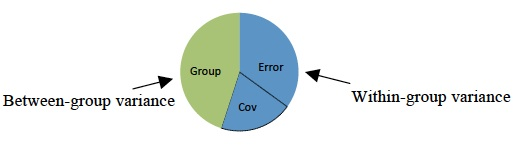# MANCOVA: Multivariate Analysis of Covariance

Share on

Hypothesis Testing > MANCOVA

< < Previous article: What is ANCOVA?

## What is MANCOVA?

MANCOVA (Multivariate Analysis of Covariance) is the multivariate counterpart of ANCOVA. MANCOVA tells you if there are statistically significant mean differences among groups. In other words, it tells you if group differences probably happened by random chance, or if there is a repeatable trend.

Watch the video for an intro:Covariates are controlled for in MANCOVA. Image: Makingstats|Wikimedia Commons

MANCOVA removes the effects of one or more covariates from your model; This allows you to see the true effect of your independent variables on your dependent variables without unwanted interference. This supercharged test comes with a cost: Required MANCOVA sample sizes are much larger than other tests. This may not be worth the extra time and expense; more often than not, a MANOVA (i.e. the same test without looking at covariates) can be more powerful.

## Types

• A one way MANCOVA needs at least four variables: One independent variable with two or more groups (levels or factors) plus two or more dependent variables and one or more covariates.
• A two way MANCOVA includes two independent variables.

## Covariates / Covariance Defined

Covariance is a measure of how much two random variables vary together. It’s similar to variance, but where variance tells you how a single variable varies, co-variance tells you how two variables vary together. A covariate can be one of these two variables. It is any variable that effects how your independent variables act upon your dependent variables. For example, confounding variables are covariates.

## Assumptions

The assumptions for MANCOVA are the same as the assumptions for MANOVA, with the addition of a couple more for covariance. As you would expect with a complex test (compared to a much simpler test like a z-test), these assumptions are lengthy and somewhat complex. This is one reason why these tests are nearly always performed using software, as most statistical software will test for these assumptions before running the test.

The covariates you choose should be correlated with the dependent variables. This can be tested prior to their inclusion in MANCOVA with correlation analysis. The best dependent variables on the other hand are not correlated with each other.

## Differences Between MANCOVA and Other Similar Tests

MANCOVA, MANOVA, ANOVA, ANCOVA: it can all get a little confusing to remember which is which. However, all of the tests can be thought of as variants of the MANCOVA, if you remember that the “M” in MANCOVA stands for Multiple and the “C” stands for Covariates. Tests can be though of as a MACOVA…

• ANOVA: … without multiple dependent variables and covariates (hence the missing M and C).
• ANCOVA: …without multiple dependent variables (hence the missing M).
• MANOVA: …without covariates (hence the missing C).

Related articles:

References:
Tabachnick, B. G., & Fidell, L. S. (1983). Using multivariate statistics. New York: Harper & Row.

CITE THIS AS:
Stephanie Glen. "MANCOVA: Multivariate Analysis of Covariance" From StatisticsHowTo.com: Elementary Statistics for the rest of us! https://www.statisticshowto.com/mancova/
---------------------------------------------------------------------------Need help with a homework or test question? With Chegg Study, you can get step-by-step solutions to your questions from an expert in the field. Your first 30 minutes with a Chegg tutor is free!